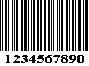## Interleaved 2 of 5 Specification

Interleaved 2 of 5 combines, or 'Interleaves' pairs of digits into a single bar code character. Each character is comprised of five bar elements and five space elements. The five bars represent the first digit of the pair, while the five spaces represent the second digit of the pair.Whether or not the elements used to encode a character are bars or spaces depends upon the location of the character within the message. The first character of the message is encoded into the bars immediately following the start character. The second character of the message is encoded into the spaces between the bars of the first character, thus eliminating the intercharacter space. Because of this, Interleaved 2 of 5 is a continuous bar code.

Due to the interleaving of the characters, the number of characters in an Interleaved 2 of 5 message must be even. The check character, if used, must be included in the character count. If the message has an odd number of characters, add a leading zero (0) to the message.

A complete Interleaved 2 of 5 symbol a quiet zone, the start character (narrow bar-narrow space-narrow bar-narrow space), the encoded data, the stop character (Wide bar-narrow space-narrow bar), and a trailing quiet zone.

## Interleaved 2 of 5 Character Set

```ASCII      Binary  Check Character
Character  Word       Value

1       10001        1
2       01001        2
3       11000        3
4       00101        4
5       10100        5
6       01100        6
7       00011        7
8       10010        8
9       01010        9
0       00110        0
Start    0000 *
Stop     100 *

NOTE: * Alternate bars and spaces, not interleaved
```

### Optional Check Digit Calculation

```Step 1: Designate the least significant (right-most)
digit postion as even (E).

5 7 6 3 5 7 9 0 1 2 5   <-- Message digits
E O E O E O E O E O E   <-- Digit positions

Step 2: Sum all the digits in the even (E) positions.

5+6+5+9+1+5 = 31

Step 3: Multiply the result by 3

31 * 3 = 93

Step 4: Sum all the digits in the odd (O) positions.

7+3+7+0+2 = 19

Step 5: Add the result of Step 2 with the result of Step 4.

93 + 19 = 112

Step 6: Subtract from the next higher multiple of 10.

120 - 112 = 8.

In this example, the check digit is 8.

Step 8: If the total length (message + check digit) is odd,
add a leading zero to the message to produce an even
number of total characters as required by the symbology.```